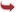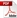International Journal of Scientific and Research Publications

#### IJSRP, Volume 6, Issue 4, April 2016 Edition [ISSN 2250-3153]Effect of linearized expressions of Langmuir equations on the prediction of the adsorption of methylene blue on rice husk
Yu-Ting Huang, Ming-Cheng Shih
Abstract: In order to investigate the effect of linearized process on the adsorption equilibrium isotherms of methylene blue from solutions onto rice husk, the experimental data were fitted to the Langmuir isotherm equations by using five different types of linear expression. The adsorption equilibrium constant related to the affinity of the binding sites and energy of adsorption (KL ) and maximum adsorption capacity (qmax) for each linear expression had been calculated. Also, values of the the amount of MB adsorbed on adsorbent at equilibrium time using the constants calculated from these five linear types of Langmuir isotherm equations were compared with the experimental data. The results indicated that the type 1 linear expressions were able to calculate the adsorption equilibrium constant and qe for the studied system (methylene blue/rice husk) well. The results also showed that it was inappropriate to use the other four proposed alternate linear expressions (type 2 to type 5) to calculate the adsorption equilibrium constant and qe for the studied system.
[VIEW FULL PAPER][DOWNLOAD]

Reference this Research Paper (copy & paste below code):

Yu-Ting Huang, Ming-Cheng Shih (2018); Effect of linearized expressions of Langmuir equations on the prediction of the adsorption of methylene blue on rice husk; Int J Sci Res Publ 6(4) (ISSN: 2250-3153). http://www.ijsrp.org/research-paper-0416.php?rp=P525300